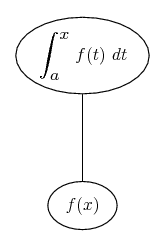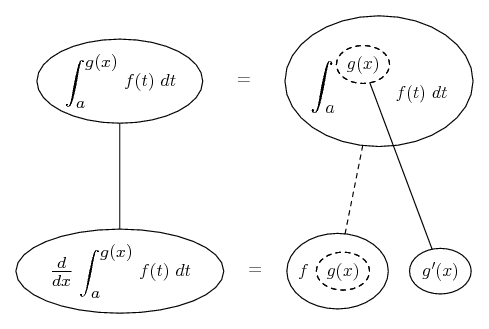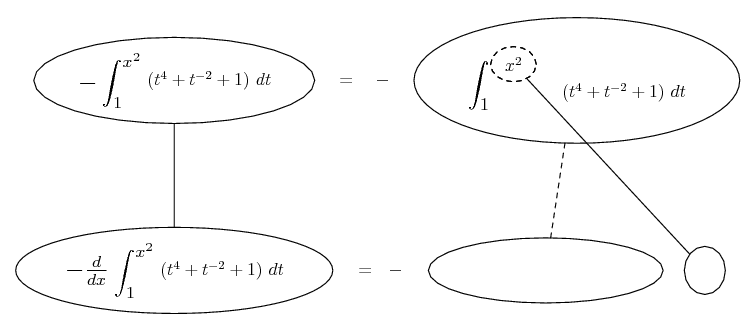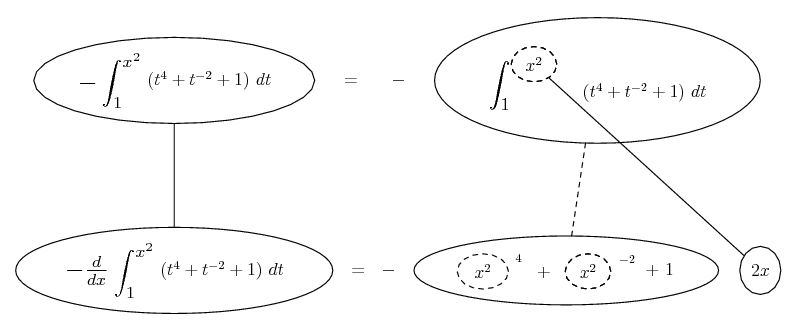# Thread: How to use chain rule on integrals

1. ## How to use chain rule on integrals

Differentiate: ∫ x^2 to 1 ( t^(4) + 1 / t^(2) + 1 )dt

I dont get how after the step:

-d/dx ∫ 1 to x^2 ( t^(4) + 1 / t^(2) + 1 )dt

how you get to: -d/du ∫ 1 to u ( t^(4) + 1 / t^(2) + 1 )dt * du/dx.

How does using the chain rule affect the upper limit? I also dont really understand the notation, since I am very used to
f'(g(x))g'(x)

2.Originally Posted by SyNtHeSiSDifferentiate: ∫ x^2 to 1 ( t^(4) + 1 / t^(2) + 1 )dt

I dont get how after the step:

-d/dx ∫ 1 to x^2 ( t^(4) + 1 / t^(2) + 1 )dt

how you get to: -d/du ∫ 1 to u ( t^(4) + 1 / t^(2) + 1 )dt * du/dx.

How does using the chain rule affect the upper limit? I also dont really understand the notation, since I am very used to
f'(g(x))g'(x)
$\displaystyle f'(x)$ is simply another notation for $\displaystyle \frac{df}{dx}$ and $\displaystyle f'(g(x))$ is the same as $\displaystyle \frac{d f(g(x))}{dx}$ so that $\displaystyle f'(g(x))g'(x)= \frac{d f(g(x))}{dg(x)}\frac{dg(x)}{dx}$.

Let $\displaystyle F(x)= \int_1^x (t^4+ t^{-2}+ 1)dt$. Then $\displaystyle F(x^2)= \int_1^{x^2} (t^4+ t^{-2}+ 1)dt$ and we are seeking $\displaystyle -\frac{dF(x^2)}{dx}$.

Let $\displaystyle u= x^2$ so that $\displaystyle F(x^2)= F(u)$. Now, the chain rule says that $\displaystyle \frac{dF}{dx}= \frac{dF}{du}\frac{du}{dx}$. Remembering that $\displaystyle F(u)= \int_1^u (t^4+ t^{-2}+ 1)dt$, that is precisely the formula you are asking about: $\displaystyle -\frac{d}{dx}\int_1^{x^2} (t^4+ t^{-2}+ 1)dt= \left(-\frac{d}{du}\int_1^u (t^4+ t^{-2}+ 1)dt\right)\frac{du}{dx}$.

3. Why do you use the chain rule since the function F(u) isnt a composite (u isnt a function composed in F). Also why isnt the function in terms of t since the function contains a "t" variable and dt?

4. t is a 'dummy variable' and often causes confusion. However, if you can just accept the rule that the lower balloon here is the derivative of the upper one with respect to x...... (which is just the FTC) then you can maybe extend to being happy with applying the chain rule. Just in case a picture helps, say... is the chain rule. Straight continuous lines differentiate downwards (integrate up) with respect to x, and the straight dashed line similarly but with respect to the dashed balloon expression (the inner function of the composite which is subject to the chain rule).

Then the FTC with chain rule is...In this case...Spoiler:BTW, correcting small typo here as Lagrange notation's ambiguity on this very point is what H of I was meaning to clarify (I think)...Originally Posted by HallsofIvy$\displaystyle f'(x)$ is simply another notation for $\displaystyle \frac{df}{dx}$ and $\displaystyle f'(g(x))$ is the same as $\displaystyle \frac{d f(g(x))}{dg(x)}$ so that $\displaystyle f'(g(x))g'(x)= \frac{d f(g(x))}{dg(x)}\frac{dg(x)}{dx}$

_________________________________________
Don't integrate - balloontegrate!

Balloon Calculus; standard integrals, derivatives and methods

Balloon Calculus Drawing with LaTeX and Asymptote!

5. Howcome you didnt sub in the lower limit and calculate F(x^2) - F(1)?

6.Originally Posted by SyNtHeSiSHowcome you didnt sub in the lower limit and calculate F(x^2) - F(1)?
You could do that (see Differentiation under the integral sign - Wikipedia, the free encyclopedia), but because the derivative of the constant (1) is zero the effect of doing so would be nil. So you can (I think) think of -F(1) as the constant of integration that disappears on the way down. I may try and amend the diagram later to make that clearer.

#### Search Tags

chain, integrals, rule